Mathematical modeling of any control system is the first and foremost task that a control engineer has to accomplish for design and analysis of any control engineering problem. It is nothing but the process or technique to express the system by a set of mathematical equations (algebraic or differential in nature). Now one may inquire– what is meant by analysis and design? How modeling of control system is related to analysis and design?

Analysis means the process of finding the response or output of a system when it is excited by an input or excitation provided we know the mathematical model of the system.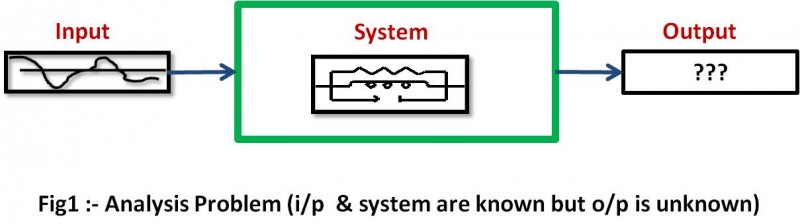On the other hand, design or synthesis means we have to find out the system equations or the arrangement of the components, provided we know the output of the system for an input. In such case the system is conceived as black box i.e. we don’t know what is inside the box and in order to find what is inside it we excite the system by different test signals and measure or observe the nature of the response.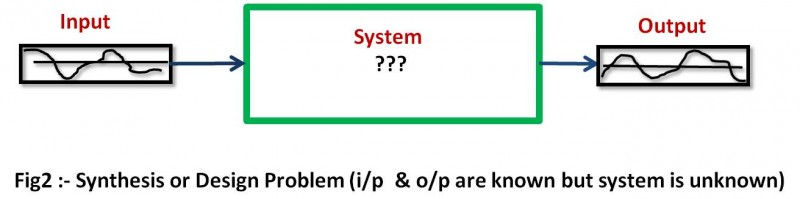Design and analysis are closely related to each other as far as the construction of a control system is concerned. A control engineer first needs to know under different input or environmental conditions how the system behaves. Based on this information he has to estimate the mathematical model of the system. Undoubtedly this task is design or synthesis of the system that we have discussed earlier. But this model can’t be directly realized using hardware without checking the fact how it behaves when it is subjected to different inputs. For this reason, the estimated model is simulated using different software to check whether the system is able to respond properly when it is excited by different inputs that the system is likely to face in real life. This task is analysis, as mentioned earlier.

This analysis shows that sometimes the system’s estimated model is unable to follow the desired output when the system is subjected to practical outputs. (In fact effect of noise and disturbances are also externally added to this analysis based on software simulation). This is because, for the sake of computational ease we often neglect some of the practical aspects and the effect of the change in operating conditions as well as the parameter variations in the estimated model of the system. Hence according to the software simulation (analysis) we have to change the proposed model of the system such that it can be properly implanted. Although control engineering has sophisticated mathematical tools and software for system design, the above process of trial and error involving both of the synthesis and analysis is still inevitable to some extent.

Methods of finding mathematical models: – One way of modeling a system is straight forward. When we know the physical components of the system, we can apply the basic laws of physics to directly obtain a set of the differential equations as a mathematical model of the system.

Other way is quite roundabout. In this method the system is treated as a black box where we can only get the outputs due to different inputs used to excite the system. From these input-output data we are to find the mathematical model of the system that it termed as system identification.

Different mathematical models:  – Commonly used mathematical models are-

1. Differential equation model.
2. State space model.
3. Transfer function model.

Use of the models depends on the application. For example, to find the transient or steady state response of SISO (Single Input Single Output) LTI (Linear Time Invariant) system transfer function model is useful. On the other hand for optimal control application state space model is useful. Usefulness of transfer function model is discussed latter.

Simplicity Vs accuracy: – Engineering is all about trade off among different conflicting requirements. Simplicity and accuracy are such two parameters. Hence judiciously they should be chosen as per specific application.

In this context it is worthwhile to mention that if any practical system is to be modeled very accurately (taking into consideration all the practical aspects to perfectly represent the behavior of the system) the model would be Time varying (i.e. parameters varies with time), Non-linear (does not satisfy linearity properties and hence the response of the system subjected to different outputs cannot be obtained by superposition principle), and Distributed parameter model. But in most of the applications such complicated models can easily be reduced into simple Time variant, Linear, Lumped models with negligible loss of accuracy and hence such approximations are very useful for mathematical analysis of the system. This is a fundamental approximation should always be kept in mind for TRANSFER Function modeling.

Differential equation model and Transfer Function model: – As a general assumption that is all ready mentioned- All the systems considered in this technique are essentially Linear, Time Invariant system (commonly known as LTI) with Lumped parameters.

By applying the laws of physics that govern the dynamics of the system we are able to find a set of differential equations. For mechanical systems such laws are Newton’s laws of motion and for electrical systems such laws are KCL & KVL. Since we have assumed that system parameters are lumped these set of equations are ordinary differential equations (ODE) and assumption of Linear System makes these equations linear in nature. Thus we finally get a set of linear ordinary differential equations for a LTI lumped parameter by applying general laws of physics.

Let’s take an example to illustrate how we can obtain the differential equation of a system. The system considered here is a mass-spring-damper system. Friction at the surface is considered and this friction acts a damping element.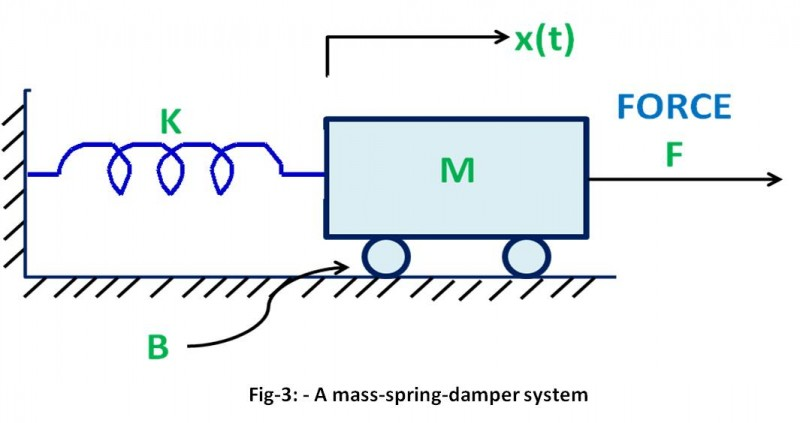M: – Mass

B: – Damping co-efficient.

K: – Spring constant.

x(t):- Displacement (from the unrestrained position of the spring).

v(t) :- Velocity.

The free body diagram is drawn-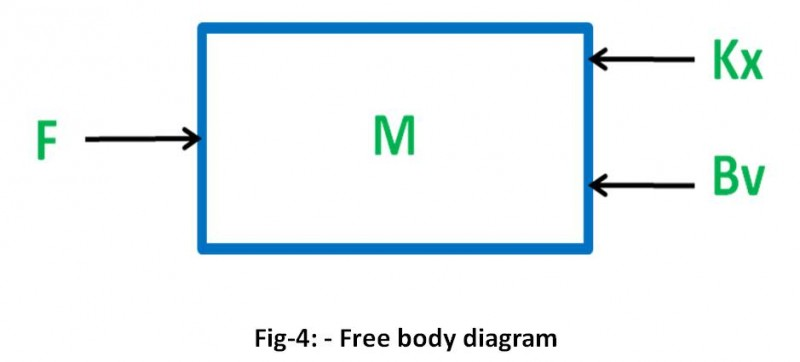Note that the equation is 2nd order differential equation. There are two energy storing elements in the system and they are spring and mass of the body. Spring acts as a Potential energy (P.E) storage element where as Mass acts as Kinetic energy (K.E) storage element. Hence this gives a very important fact in general the no of the energy storing elements is equal to the order of the system.

Now we take an example of an electrical system -a simple RLC series network.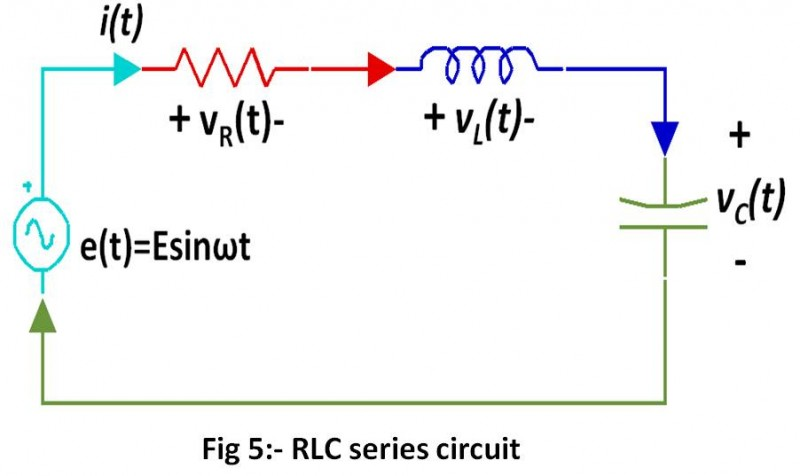By applying KCL and KVL we get-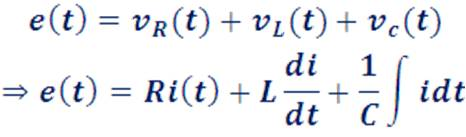Differentiating both sides with respect to t we get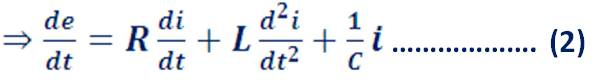Like the previous example of mechanical system here also we find since there are 2 energy storing elements (Inductor and Capacitor) in the electrical system, the order of the ODE governing system dynamics is 2.

Transfer function model: – One problem of working with equation (1) and (2) is the fact that they are differential in nature. Handling and solving differential equations are quite cumbersome as compared to linear algebraic equations. What would happen if we would have to deal with linear algebraic equations in lieu of differential equations? The answer is- Simply Great! But is there anything to convert these tiresome differential equations into linear algebraic equations? The answer is –YES! And that is Laplace Transformation. It is defined as follows-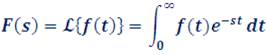Note that Laplace Transformation of f(t) is denoted by   which is essentially a function of ‘s’ and hence it is also written as F(s). Look an interesting fact – the ‘st’ is dimensionless quantity (that is a pure number with no unit) as it is the exponent of a number (e). Since the dimension of ‘t’ is ‘time’ , undoubtedly the dimension of ‘s’ is  ‘1/time’ which is nothing but ‘frequency’. Thus, if ‘t’ is measured in ‘second’ the unit of ‘f’ should be ‘Hz’. This is the reason that people say Laplace Transformation is an integral transformation from time domain to frequency domain.

We have just defined Laplace Transformation and haven’t shown its usefulness. We are going to use Laplace Transformation to ease our computational labor and this is the rudimentary fact which will form the foundation of Transfer Function modeling. Just carefully look at the following equations-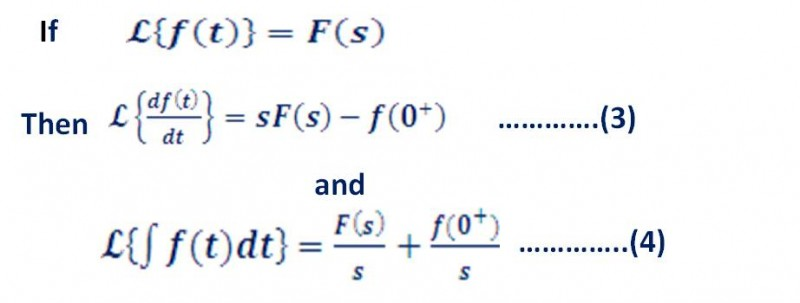[Here the proofs of the results are avoided for those who don’t like much math. Sorry Math lovers!!! I have to avoid the romantic fantasy of deriving such useful results for you!! You are requested to go through any text on Laplace Transformation.]

It can be easily seen that the differentiation in time domain is realized in Frequency or ‘s’ domain by multiplication by ‘s’ and similarly the integration is realized as division by ‘s’. This is a very important result which helps us to think frequency domain representation as an easy alternative of time domain in certain simple situations as far as the computational simplicity is concerned.

What are those simple situations? In equation (3) and (4) there are terms f(0) which physically represents the initial energy storage of the energy storing elements. It is termed as initial condition. For example it may refer to the initial charge stored in Capacitor (C) or initial current through an Inductor (L). Although while solving networks problems (especially transient) we do consider these initial conditions but in case of TRANSFER FUNCTION modeling we neglect these initial conditions or in other words we assume that the initial conditions are zero, which is also sometimes termed as the ‘initially relaxed system’. This is another important assumption for Transfer Function modeling.

Now we will try to realize equation (1) and (2) in frequency domain.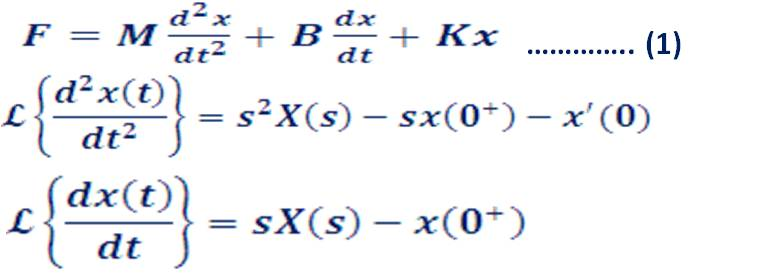Now, by assuming zero initial condition we get, x(0+)=0  and x’(0)=0and hence we can straight way write the equation (1) in the following form-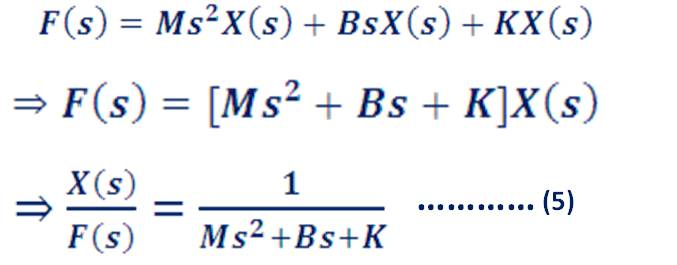It is the transfer function model of the mechanical system (Fig-1 ) dictated by equation (5).

Transfer functions are normally denoted by the symbols G(s) or H(s).

Hence Transfer Function G(s)=X(s)/F(s) which is defined as the ratio  of the  excitation  variable to the response variable in ‘s’ domain  for a LTI system with all initial conditions set equal to zero. [Here Force F(s) is the excitation variable and Displacement X(s) is the response variable.]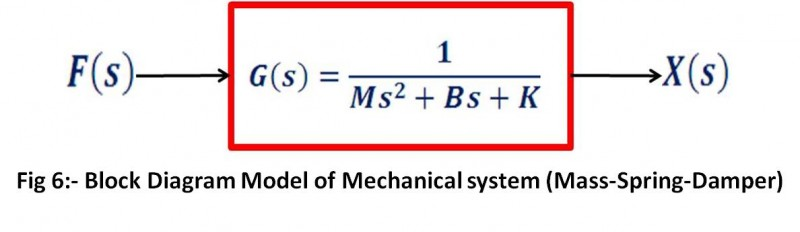Equation (2) can also be converted into Transfer Function: –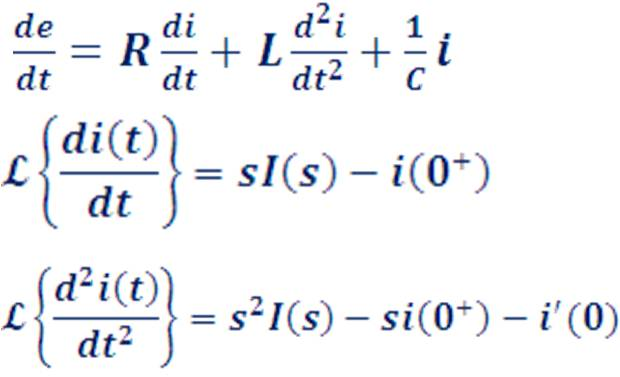Again by setting all the initial conditions to zero and writing equation (2) in frequency domain we get: –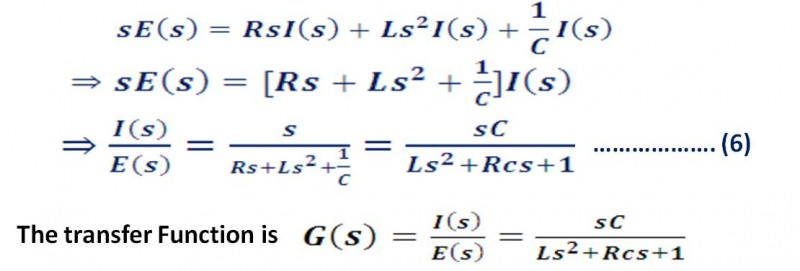Clearly the Transfer Function model of the electrical system (Fig – 3) is given by equation (6).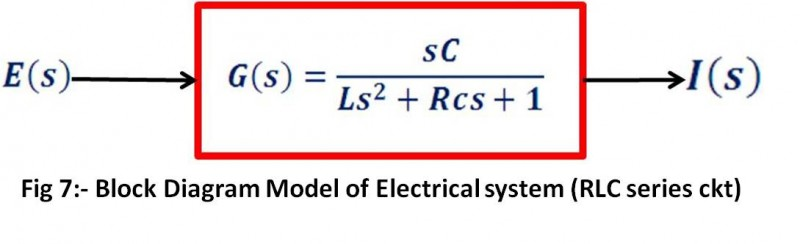Share.